Shivani Sharma — August 7, 2021

This article was published as a part of the Data Science Blogathon

## Introduction

This article aims to compare four different deep learning and machine learning algorithms to build a spam detector and evaluate their performances. The dataset we used was from a shuffled sample of email subjects and bodies containing both spam and ham emails in numerous proportions, which we converted into lemmas. Email Spam Detection is one of the most effective projects of Deep learning but this is often also one project where people lose their confidence to search out the simplest model for accuracy purposes. In this article, we are going to detect the spam in the mail using four different techniques and compare them to get the most accurate model.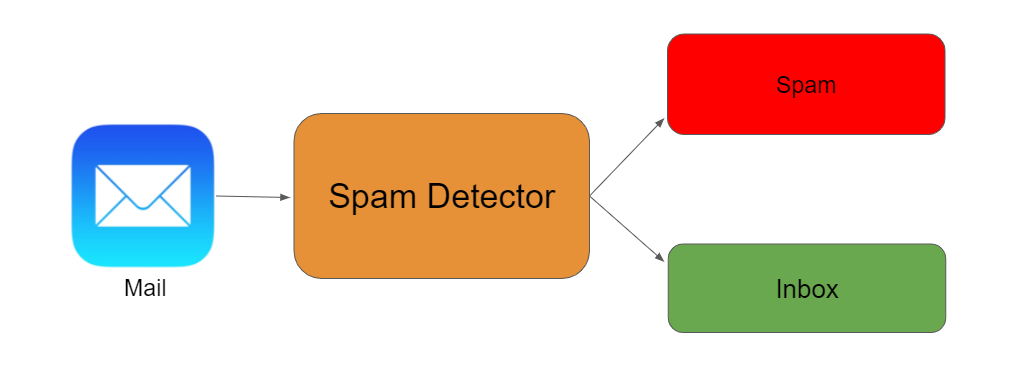Source

## WHY SPAM DETECTION?

An email has become one of the foremost important kinds of communication. In 2014, there are estimated to be 4.1 billion email accounts worldwide, and about 196 billion emails are sent day after day worldwide. Spam is one of the main threats posed to email users. All email flows that were spam in 2013 are 69.6%. Therefore, an effective spam filtering technology is a significant contribution to the sustainability of cyberspace and our society. As the importance of email is not lesser than your bank account containing 1Cr., then protecting it from spam or frauds is also mandatory.

## Data Preparation

To prepare the data, we followed the steps below:

2. Read the mbox files into lists using the ‘mailbox’ package. Each element in the list contained an individual email. In the first iteration, we included 1000 ham mails and 400 spam mails (we tried different ratios after the first iteration).

3. Unpacked each email and concatenated their subject and body. We decided to include the email subject as well in our analysis because it is also a great indicator of whether an email is a spam or ham.

4. Converted the lists to data frames, joined the spam and ham data frames, and shuffled the resultant data frame.

5. Split the data frame into train and test data frames. The test data was 33% of the original dataset.

6. Split the mail text into lemmas and applied TF-IDF transformation using CountVectorizer followed by TF-IDF transformer.

7. Trained four models using the training data:

• Naive Bayes
• Decision Trees
• Support Vector Machine (SVM)
• Random Forest

8. Using the trained models, predicted the email label for the test dataset. Calculated four metrics to gauge the performance of the models as Accuracy, Precision, Recall, F-score, AUC.

## CODE

#### 1.Importing the libraries

```#import all the needed libraries
import mailbox
%matplotlib inline
import matplotlib.pyplot as plt
import csv
from textblob import TextBlob
import pandas
import sklearn
#import cPickle
import numpy as np
from sklearn.feature_extraction.text import CountVectorizer, TfidfTransformer
from sklearn.naive_bayes import MultinomialNB
from sklearn.svm import SVC, LinearSVC
from sklearn.metrics import classification_report, f1_score, accuracy_score, confusion_matrix
from sklearn.pipeline import Pipeline
from sklearn.grid_search import GridSearchCV
from sklearn.cross_validation import StratifiedKFold, cross_val_score, train_test_split
from sklearn.tree import DecisionTreeClassifier
from sklearn.learning_curve import learning_curve
#import metrics libraries
from sklearn.metrics import confusion_matrix
from sklearn.metrics import precision_score
from sklearn.metrics import recall_score
from sklearn.metrics import f1_score
from sklearn.metrics import roc_auc_score```

#### 2.Function to get email text from email body

```def getmailtext(message): #getting plain text 'email body'
body = None
#check if mbox email message has multiple parts
if message.is_multipart():
for part in message.walk():
if part.is_multipart():
for subpart in part.walk():
if subpart.get_content_type() == 'text/plain':
elif part.get_content_type() == 'text/plain':
#if message only has a single part
elif message.get_content_type() == 'text/plain':
#return mail text which concatenates both mail subject and body
mailtext=str(message['subject'])+" "+str(body)
return mailtext```

#### 3. Read spam m-box email file

```mbox = mailbox.mbox('Spam.mbox')

mlist_spam = []
#create list which contains mail text for each spam email message
for message in mbox:
mlist_spam.append(getmailtext(message))
#break
mbox_ham = mailbox.mbox('ham.mbox')

mlist_ham = []
count=0
#create list which contains mail text for each ham email message
for message in mbox_ham:

mlist_ham.append(getmailtext(message))
if count>601:
break
count+=1```

#### 4. Creating two datasets from spam/ham emails containing information like mail text, mail label, and mail length

```#create 2 dataframes for ham spam mails which contain the following info-
#Mail text, mail length, mail is ham/spam label
import pandas as pd
spam_df = pd.DataFrame(mlist_spam, columns=["message"])
spam_df["label"] = "spam"

spam_df['length'] = spam_df['message'].map(lambda text: len(text))

ham_df = pd.DataFrame(mlist_ham, columns=["message"])
ham_df["label"] = "ham"

ham_df['length'] = ham_df['message'].map(lambda text: len(text))5.Function to apply BOW and TF-IDF transforms

```def features_transform(mail):
#get the bag of words for the mail text
bow_transformer = CountVectorizer(analyzer=split_into_lemmas).fit(mail_train)
#print(len(bow_transformer.vocabulary_))
messages_bow = bow_transformer.transform(mail)
#print sparsity value
print('sparse matrix shape:', messages_bow.shape)
print('number of non-zeros:', messages_bow.nnz)
print('sparsity: %.2f%%' % (100.0 * messages_bow.nnz / (messages_bow.shape * messages_bow.shape)))
#apply the TF-IDF transform to the output of BOW
tfidf_transformer = TfidfTransformer().fit(messages_bow)
messages_tfidf = tfidf_transformer.transform(messages_bow)
#print(messages_tfidf.shape)
#return result of transforms
return messages_tfidf```

#### 6. Function to print the associated model performance metrics

```#function which takes in y test value and y predicted value and prints the associated model performance metrics
def model_assessment(y_test,predicted_class):
print('confusion matrix')
print(confusion_matrix(y_test,predicted_class))
print('accuracy')
print(accuracy_score(y_test,predicted_class))
print('precision')
print(precision_score(y_test,predicted_class,pos_label='spam'))
print('recall')
print(recall_score(y_test,predicted_class,pos_label='spam'))
print('f-Score')
print(f1_score(y_test,predicted_class,pos_label='spam'))
print('AUC')
print(roc_auc_score(np.where(y_test=='spam',1,0),np.where(predicted_class=='spam',1,0)))
plt.matshow(confusion_matrix(y_test, predicted_class), cmap=plt.cm.binary, interpolation='nearest')
plt.title('confusion matrix')
plt.colorbar()
plt.ylabel('expected label')
plt.xlabel('predicted label')```

Let’s begin the comparative analysis of four different models to get the highest-performing algorithm.

### 1.Naive Bayes Model

Naive Bayes with a bag of words approach using TF-IDFNaive Bayes is that the simplest classification algorithm (fast to form, regularly used for spam detection). it is a popular (baseline) method for text categorization, the matter of judging documents as belonging to 1 category or the opposite (such as spam or legitimate, sports or politics, etc.) with word frequencies due to the features.

Feature extraction using BOW:

TF-IDFTerm frequency-Inverse document frequency uses all the tokens within the dataset as vocabulary. The term frequency and the number of documents during which token occurs are responsible for determining the Inverse document frequency. What this ensures is that, if a token occurs frequently during a document that token will have high TF but if that token occurs frequently within the bulk of documents then it reduces the IDF. Both these TF and IDF matrices for a selected document are multiplied and normalized to make the TF-IDF of a document.

CODE

```#create and fit NB model
modelNB=MultinomialNB()
modelNB.fit(train_features,y_train)
#transform test features to test the model performance
test_features=features_transform(mail_test)
#NB predictions
predicted_class_NB=modelNB.predict(test_features)
#assess NB
model_assessment(y_test,predicted_class_NB)```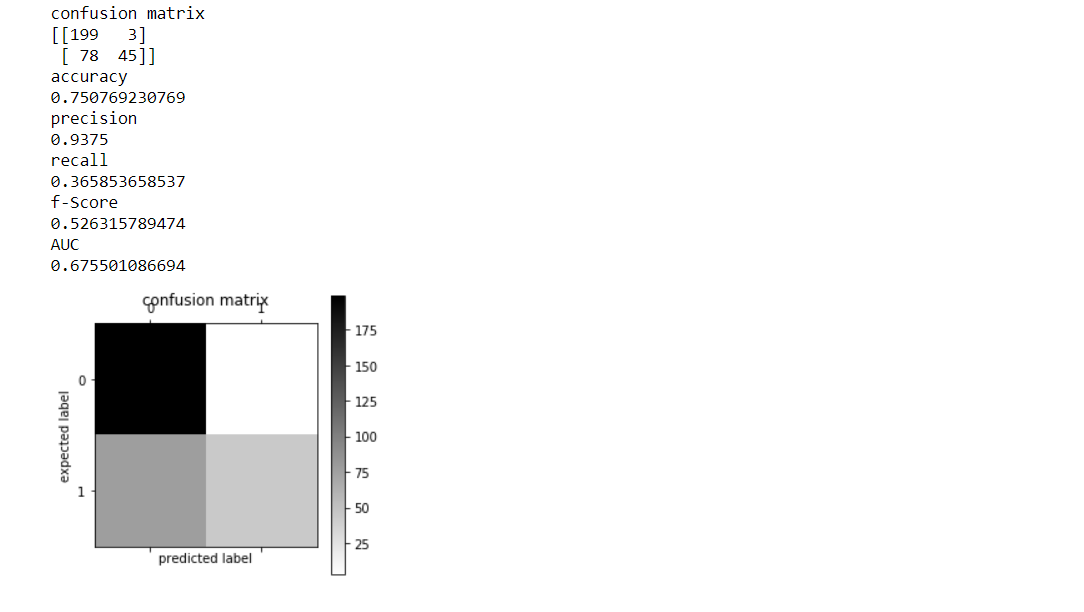### 2.Decision Tree Model

Decision trees are used for classification and regression. The theory might be a measure to define this degree of disorganization during a system called Entropy. The entropy factor varies from sample to sample. The entropy is zero for the homogeneous sample, and for the equal dividend sample, the entropy is 1. It chooses the split which has rock bottom entropy compared to the parent node and other splits. The lesser the entropy, the upper it is.

#### CODE

```#create and fit tree model
model_tree=DecisionTreeClassifier()
model_tree.fit(train_features,y_train)
#run model on test and print metrics
predicted_class_tree=model_tree.predict(test_features)
model_assessment(y_test,predicted_class_tree)```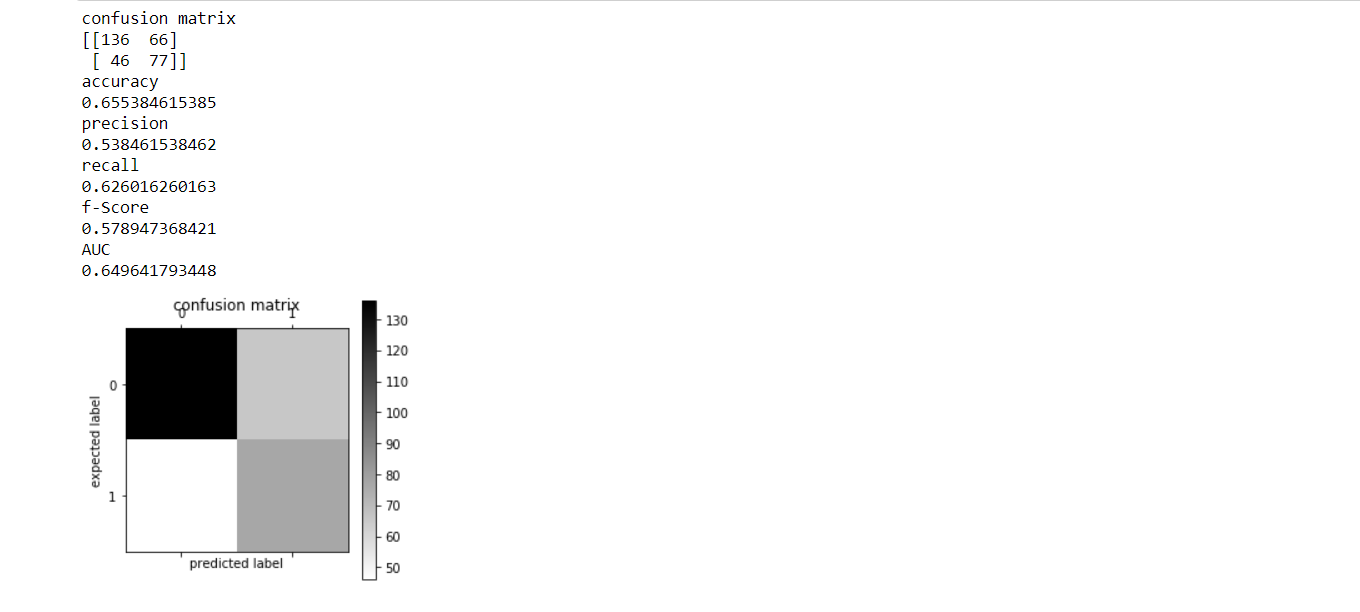### 3. Support Vector Machine

Both the classification or regression challenges are working perfectly for this well-known supervised machine learning algorithm(SVM). However, it’s mostly employed in classification problems. When we are working with this algorithm, In n-dimensional space, we are going to plot each data item to some extent such that the worth of every feature being the worth of a selected coordinate. Support Vector Machine could even be a frontier that best segregates the 2 classes (hyper-plane/ line).

CODE

```#create and fit SVM model
model_svm=SVC()
model_svm.fit(train_features,y_train)
#run model on test and print metrics
predicted_class_svm=model_svm.predict(test_features)
model_assessment(y_test,predicted_class_svm)```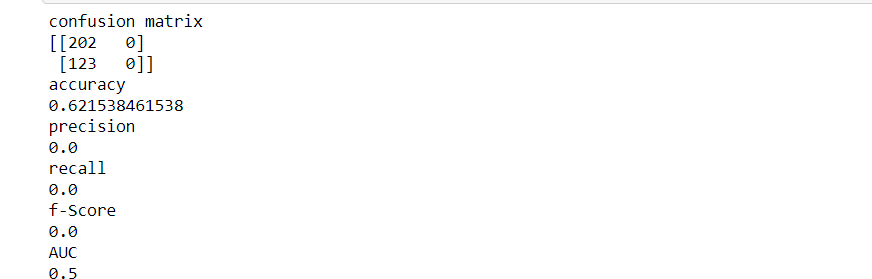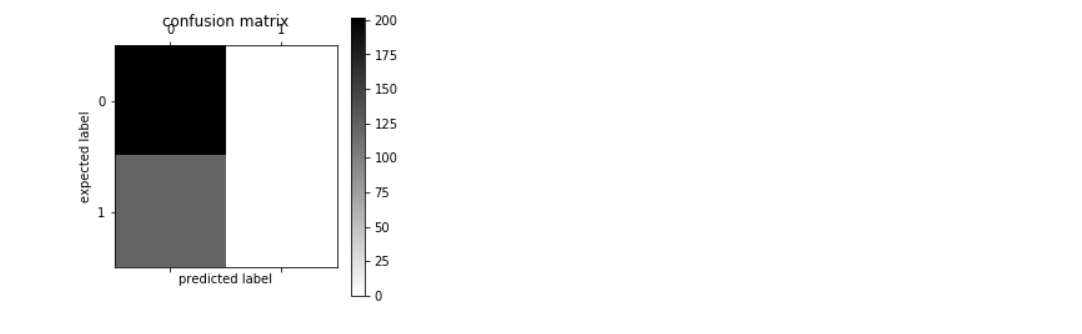### 4. Random Forest

Random forest is like bootstrapping algorithm with a call tree (CART) model. The last word prediction might be a function of each prediction. This final prediction can simply be the mean of every prediction. Random forest gives rather more accurate predictions when put next to simple CART/CHAID or regression models in many scenarios. These cases generally have a high number of predictive variables and an enormous sample size. this is often actually because it captures the variance of several input variables at a uniform time and enables a high number of observations to participate within the prediction.

CODE

```from sklearn.ensemble import RandomForestClassifier
#create and fit model
model_rf=RandomForestClassifier(n_estimators=20,criterion='entropy')
model_rf.fit(train_features,y_train)
#run model on test and print metrics
predicted_class_rf=model_rf.predict(test_features)
model_assessment(y_test,predicted_class_rf)```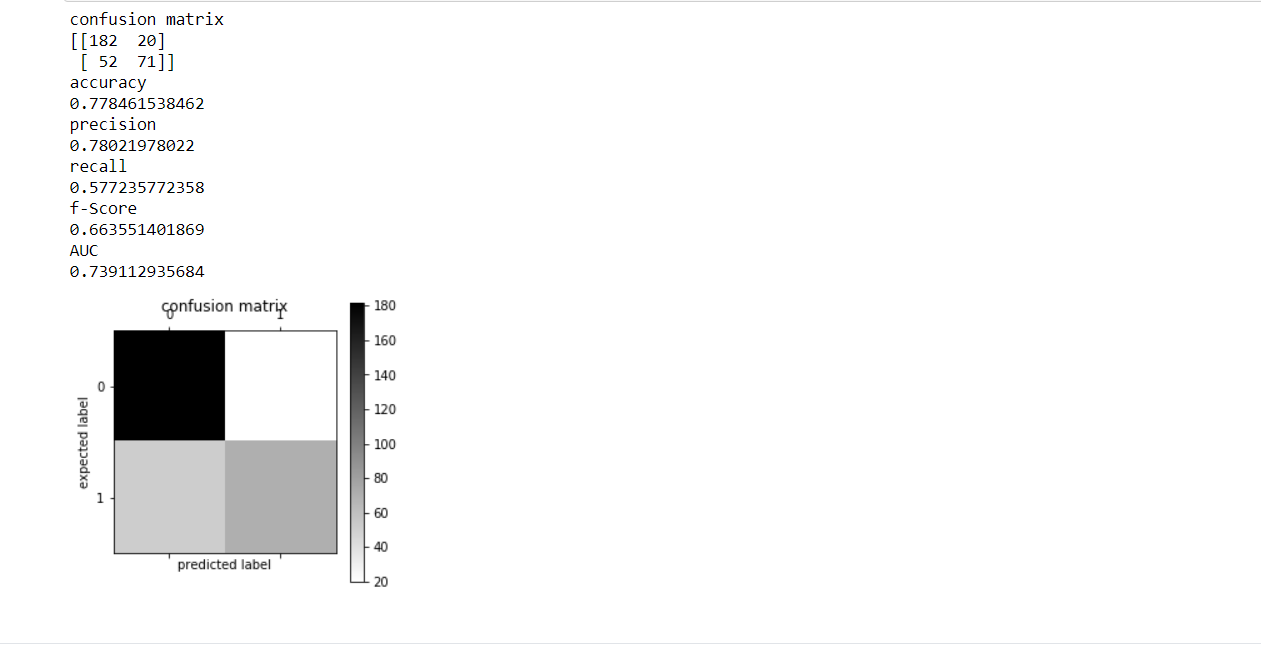## COMPARISON:-

As you see the output of all 4 models you can easily compare and find their accuracy. According to the above explanation the decreasing order of accuracy is depicted as:

MODEL                                                            ACCURACY

RANDOM FOREST                                        0.77846

NAIVE BAYES                                                0.75076

DECISION TREE MODEL                              0.65538

SUPPORT VECTOR MACHINE                    0.62153

RESULTS

The results are highly clear that Random Forest is the most accurate method while detecting spam emails. The reason for the same is its ability of wide diversion to find the best feature using its randomness. The model that can’t be used for such email spam detection is SVM. The reason for the same is its small expansion. SVM can’t have the ability to handle huge data.

## CONCLUSION

This article will help you in the implementation of a spam detection project with the help of deep learning. This is highly based on a comparative analysis of four different models. Stay tuned on Analytics Vidya for upcoming articles. You can use this as a reference. Don’t hesitate to put your inputs in the below chatbox. You can also ping me on LinkedIn at https://www.linkedin.com/in/shivani-sharma-aba6141b6/